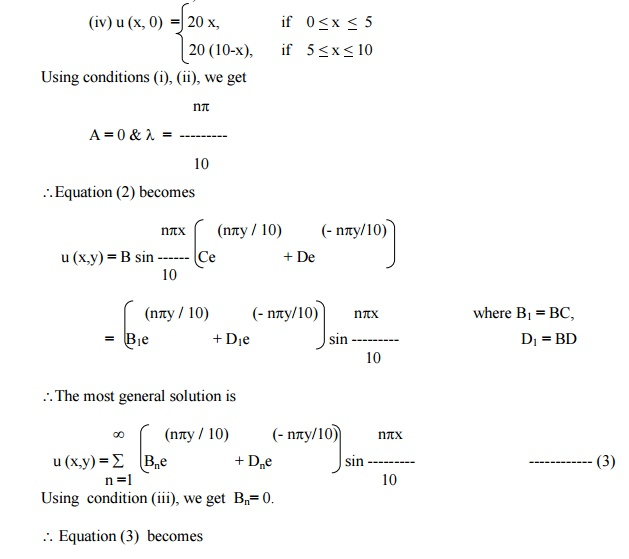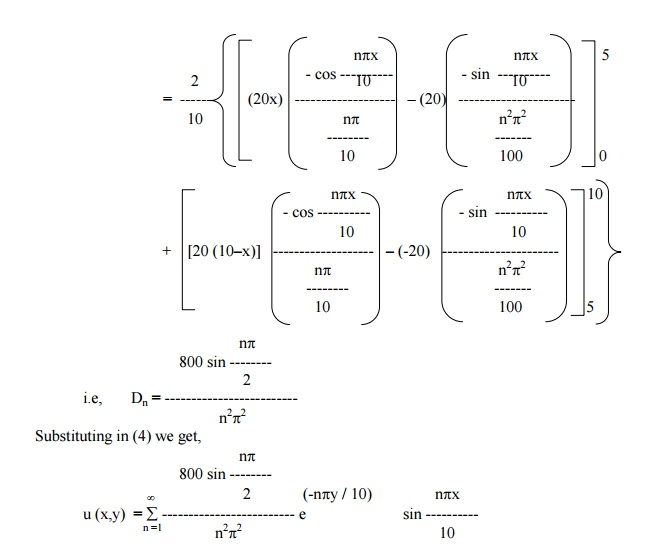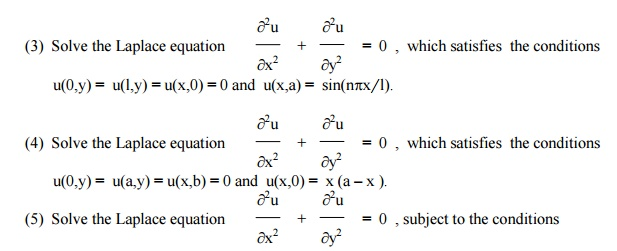Home | | Transforms and Partial Differential Equations | Solution of LaplaceŌĆÖs equation (Two dimensional heat equation)

# Solution of LaplaceŌĆÖs equation (Two dimensional heat equation)

Let u = X(x) . Y(y) be the solution of (1), where ŌĆ×XŌĆ¤ is a function of ŌĆ×xŌĆ¤ alone and ŌĆ×YŌĆ¤ is a function of ŌĆ×yŌĆ¤ alone.

Solution of LaplaceŌĆÖs equation  (Two dimensional heat equation)

The Laplace equation isLet u = X(x) . Y(y) be the solution of (1), where ŌĆ×XŌĆ¤ is a function of ŌĆ×xŌĆ¤ alone and ŌĆ×YŌĆ¤ is  a function of ŌĆ×yŌĆ¤ alone.Now the left side of (2) is a function of ŌĆ×xŌĆ¤ alone and the right side is a function of ŌĆ×tŌĆ¤ alone. Since ŌĆ×xŌĆ¤ and ŌĆ×tŌĆ¤ are independent variables, (2) can be true only if each side is equal to a constant.Solving equations (3), we get

(i) when ŌĆ×kŌĆ¤ is positive and k = l2, say

X = c1 elx  + c2  e - lx

Y = c3 cosly + c4 sin ly(iii)               when   ŌĆ×kŌĆ¤   is   zero.

X = c9  x + c10

Y = c11 x + c12

Thus the various possible solutions of (1) areOf these three solutions, we have to choose that solution which suits the physical nature of the problem and the given boundary conditions.

Example 12

An infinitely long uniform plate is bounded by two parallel edges x = 0 & x = Ōäō and an end at right angles to them. The breadth of this edge y = 0 is Ōäō and this edge is maintained at a temperature f (x). All the other 3 edges are at temperature zero. Find the steady state temperature at any interior point of the plate.

Solution

Let u (x,y) be the temperature at any point x,y of the plate.

Also u (x,y) satisfies the equationUsing (7) in (5), we get the required solution.

Example 13

A rectangular plate with an insulated surface is 8 cm. wide and so long compared to its width that it may be considered as an infinite plate. If the temperature along short edge y = 0 is u(x,0) = 100 sin (px/8), 0 < x < 8, while two long edges x = 0 & x = 8 as well as the other short edges are kept at 0┬░C. Find the steady state temperature at any point of the plate.

Solution

The two dimensional heat equation is given byThe boundary conditions are

(i) u (0, y) = 0,      for 0 < y < ┬ź

(ii) u (8, y) = 0,     for 0 < y < ┬ź

(iii) u (x, ┬ź) = 0,   for 0 < x < 8

(iv) u (x, 0) = 100 Sin (┬źx/8,) for 0 < x < 8

Using conditions (i), & (ii), we getComparing like coefficients on both sides, we get

D1 = 100, D2 = D3 = . . . . = 0

Substituting in (4), we get

u (x,y) = 100 e(-py / 8)     sin (px / 8)

Example 14

A rectangular plate with an insulated surface 10 c.m wide & so long compared to its width that it may considered as an infinite plate. If the temperature at the short edge y = 0 is given byand all the other 3 edges are kept at temperature 0┬░C. Find the steady state temperature at any point of the plate.

Solution

The temperature function u (x,y) is given by the equationThe boundary conditions are

(i) u (0, y)   = 0,             for 0 < y < ┬ź

(ii) u (10, y) = 0,            for 0 < y < ┬ź

(iii) u (x, ┬ź) = 0,            for 0 < x  < 10Example 15

A rectangular plate is bounded by the lines x = 0, x = a, y = 0 & y = b. The edge temperatures are u (0,y) = 0, u (x,b) = 0, u (a,y) = 0 & u (x,0) = 5 sin (5px / a) + 3 sin (3px / a). Find the steady state temperature distribution at any point of the plate.

The temperature function u (x,y) satisfies the equationThe boundary conditions are

(i) u (0,y) = 0,                          for 0 < y < b

(ii) u (a,y) = 0,                         for 0 < y < b

(iii) u (x, b) = 0,    for 0 < x < a

(iv) u (x,0) = 5 sin (5px / a) + 3 sin (3px / a),       for 0 < x < a.Exercisesii.           u(a,y) = 0 for 0 <<b

iii.           u(x,b) = 0 for 0 <<a

iv.          u(x,0) = sin3(px/ a) ,0 <<a.

(2)   Find the steady temperature distribution at points in a rectangular plate with insulated faces and the edges of the plate being the lines x = 0, x = a, y = 0 and y = b. When three  of the edges are kept at temperature zero and the fourth at a fixed temperature ao C.i.           u(0,y) = 0, 0 ┬Ż┬Żl   ii.   u(l,y) = 0, 0 ┬Ż┬Ż

iii.         u(x,0) = 0, 0 ┬Ż┬Żl iv.  u(x,l) = f(x), 0 ┬Ż┬Żl

(6)   A square plate is bounded by the lines x = 0, y = 0, x = 20 and y = 20. Its faces are insulated.

The temperature along the upper horizontal edge is given by u(x,0) = x (20 ŌĆōx), when 0 < x <20,

while other three edges are kept at 0o C. Find the steady state temperature in the plate.

(7) An infinite long plate is bounded plate by two parallel edges and an end at right

angles to them.The breadth is p. This end is maintained0ŌĆ¤atat a c all points and the other edges are at zero temperature. Find the steady state temperature at

any point (x,y) of the plate.

(8) An infinitely long uniform plate is bounded by two parallel edges x = 0 and x = l, and

an end at right angles to them. The breadth of this edge y = 0 is ŌĆ×lŌĆ¤ and temperature f(x). All the other three edges are at temperature zero. Find the steady state

temperature at any interior point of the plate.

(9) A rectangular plate with insulated surface is 8 cm. wide and so long compared to its width that it may be considered infinite in length without introducing an appreciable error. If the temperature along one short edge y = 0 is given by u(x,0) = 100 sin(px/ 8), 0 <<8, while the two long edges x = 0 and x = 8 as well as the other short edge are kept at 0o C, show that the steady state temperature at any point of the plane is given by u(x,y) = 100 e-py/ 8 sin px/ 8 .

(10) A rectangular plate with insulated surface is 10 cm. wide and so long compared to its width that it may be considered infinite length. If the temperature along short edge y = 0 is given

u(x,0) = 8 sin(px/ 10) when 0 <<10, while the two long edges x = 0 and x = 10 as well as the other short edge are kept at 0o C, find the steady state temperature distribution u(x,y).

Study Material, Lecturing Notes, Assignment, Reference, Wiki description explanation, brief detail
Mathematics (maths) : Applications of Partial Differential Equations : Solution of LaplaceŌĆÖs equation (Two dimensional heat equation) |